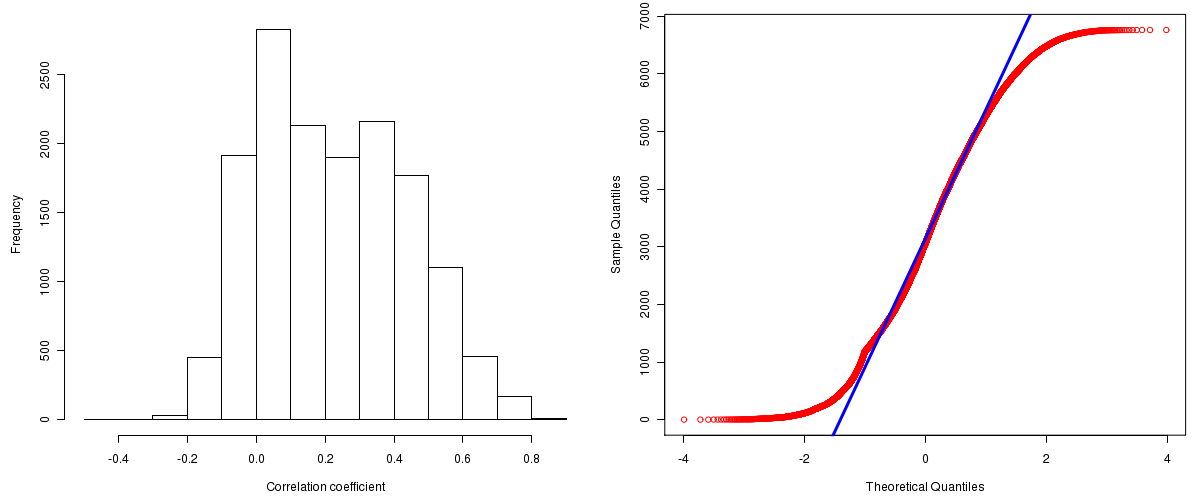Colon/Rectal Adenocarcinoma: Correlations between copy number and mRNA expression
Maintained by John Zhang (Dana-Farber Cancer Institute)
Overview
Introduction

A TCGA sample is profiled to detect the copy number variations and expressions of genes. This pipeline attempts to correlate copy number and expression data of genes across samples to determine if the copy number variations also result in differential expressions. This report contains the calculated correlation coefficients based on measurements of genomic copy number (log2) values and intensity of the expressions of the corresponding feature across patients. High positive/low negative correlation coefficients indicate that genomic alterations result in differences in the expressions of mRNA the genomic regions transcribe.

Summary

The correlation coefficients in 10, 20, 30, 40, 50, 60, 70, 80, 90 percentiles are -0.0361, 0.0211, 0.07188, 0.1326, 0.2056, 0.28476, 0.3556, 0.4269, 0.51814, respectively.

Results
Correlation results

Number of genes and samples used for the calculation are shown in Table 1. Figure 1 shows the distribution of calculated correlation coefficients and quantile-quantile plot of the calculated correlation coefficients against a normal distribution. Table 2 shows the top 20 features ordered by the value of correlation coefficients.

Table 1.  Counts of mRNA and number of samples in copy number and expression data sets and common to both

Category Copy number Expression Common
Sample 564 224 207
Genes 29390 17815 15551

Figure 1.  Summary figures. Left: histogram showing the distribution of the calculated correlations across samples for all Genes. Right: QQ plot of the calculated correlations across samples. The QQ plot is used to plot the quantiles of the calculated correlation coefficients against that derived from a normal distribution. Points deviating from the blue line indicate deviation from normality.Table 2.  Get Full Table Top 20 features (defined by the feature column) ranked by correlation coefficients

feature r p-value q-value chrom start end geneid
PSMF1 0.8342 0 0 20 1041939 1095971 9491
CDC16 0.8275 0 0 13 114018476 114056252 8881
CUL4A 0.816 0 0 13 112911087 112967393 8451
DSTN 0.8113 0 0 20 17498599 17536652 11034
YTHDF1 0.8109 0 0 20 61297226 61317983 54915
CRNKL1 0.8088 0 0 20 19963012 19984690 51340
RPL19 0.8086 0 0 17 34610062 34614506 6143
DPM1 0.8081 0 0 20 48984812 49008467 8813
CSNK2A1 0.8063 0 0 20 411338 472482 1457
TMTC4 0.8018 0 0 13 100054190 100092488 84899
POLR3F 0.801 0 0 20 18396033 18413287 10621
SAP18 0.799 0 0 13 20612685 20620977 10284
MKKS 0.7957 0 0 20 10333832 10362866 8195
DYNLRB1 0.7937 0 0 20 32567865 32592423 83658
LNX2 0.7917 0 0 13 27018050 27053940 222484
GTF2E2 0.7908 0 0 8 30555573 30635274 2961
C20orf43 0.7903 0 0 20 54477092 54527345 51507
C17orf37 0.7877 0 0 17 35138935 35140314 84299
SNRPB2 0.7875 0 0 20 16658629 16670037 6629
MED4 0.7871 0 0 13 47548093 47567241 29079
Methods & Data
Input

Gene level (TCGA Level III) expression data and copy number data of the corresponding loci derived by using the CNTools package of Bioconductor were used for the calculations. Pearson correlation coefficients were calculated for each pair of genes shared by the two data sets across all the samples that were common.

Correlation across sample

Pairwise correlations between the log2 copy numbers and expressions of each gene across samples were calculated using Pearson correlation.